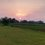# Oh boy! Can't tell you the limit

I guess everyone seems to be knowing the old results,

$\Large\bullet \lim_{x\to 0}\frac{\sin x}{x}=1$ $\Large\bullet \lim_{x\to 0}\frac{\tan x}{x}=1$ $\Large\bullet \lim_{x\to 0}[\frac{\sin x}{x}]=0$ $\Large\bullet \lim_{x\to 0}[\frac{\tan x}{x}]=1$ $$(\textbf {[•] is greatest integer function})$$

Yeah you know them, great. One can easily get them straight from the inequality

$\sin x for $0< x<\frac{π}{2}$

Also it can even be realized by the graphs of $\sin x$ & $\tan x$ that as $x$ comes closer to origin (or$x\to 0$), the graphs of these trigonometric functions $\color{#3D99F6}{\textbf {coincide the line y=x}}$, thereby giving the first two results in our hand.

Furthermore it's seen that near the origin, the sin curve $\color{#3D99F6}{\textbf { is slightly below the line y=x}}$ & tan curve $\color{#3D99F6}{\textbf { is slightly above the line y=x}}$. Did you smell the proof of the last two of the old results?

Anyways, everything's real easy till we reach to the puzzle of ours.. $\Large\text{What's the value of} \color{#D61F06} { \lim_{x\to 0}[\frac{\sin x•\tan x}{x^2}]}$ Can you submit the result decorated with the rigorous proof of yours??Note by Sanjeet Raria
6 years, 9 months ago

This discussion board is a place to discuss our Daily Challenges and the math and science related to those challenges. Explanations are more than just a solution — they should explain the steps and thinking strategies that you used to obtain the solution. Comments should further the discussion of math and science.

When posting on Brilliant:

• Use the emojis to react to an explanation, whether you're congratulating a job well done , or just really confused .
• Ask specific questions about the challenge or the steps in somebody's explanation. Well-posed questions can add a lot to the discussion, but posting "I don't understand!" doesn't help anyone.
• Try to contribute something new to the discussion, whether it is an extension, generalization or other idea related to the challenge.

MarkdownAppears as
*italics* or _italics_ italics
**bold** or __bold__ bold
- bulleted- list
• bulleted
• list
1. numbered2. list
1. numbered
2. list
Note: you must add a full line of space before and after lists for them to show up correctly
paragraph 1paragraph 2

paragraph 1

paragraph 2

[example link](https://brilliant.org)example link
> This is a quote
This is a quote
    # I indented these lines
# 4 spaces, and now they show
# up as a code block.

print "hello world"
# I indented these lines
# 4 spaces, and now they show
# up as a code block.

print "hello world"
MathAppears as
Remember to wrap math in $$ ... $$ or $ ... $ to ensure proper formatting.
2 \times 3 $2 \times 3$
2^{34} $2^{34}$
a_{i-1} $a_{i-1}$
\frac{2}{3} $\frac{2}{3}$
\sqrt{2} $\sqrt{2}$
\sum_{i=1}^3 $\sum_{i=1}^3$
\sin \theta $\sin \theta$
\boxed{123} $\boxed{123}$

Sort by:

is it 1 ?

- 6 years, 9 months ago

Yes indeed.. But share the proof.

- 6 years, 9 months ago

use expansion of sinx and tanx

- 6 years, 9 months ago

Since this is a even function, it suffice to check

$\lim_{x \rightarrow 0^{+} } [ \frac{\sin(x)\tan(x)}{x^2} ]$

Consider the function $f(x) = \sin(x)\tan(x)-x^2 \\ f'(x) = \sec(x)\tan(x) + \sin(x) - 2x \ge 2\tan(x)-2x \ge 0$(BY AM-GM inequality. So this function is increasing. This means that, $f(0^{+}) > f(0)= 0$ So $\frac{\sin(x)\tan(x)}{x^2} \ge 1$ . Also we see that $\lim_{x \rightarrow 0^{+} } \frac{\sin(x)\tan(x)}{x^2} = 1$ . So combining these two gives, $\lim_{x \rightarrow 0} [ \frac{\sin(x)\tan(x)}{x^2} ] = 1$

- 6 years, 9 months ago